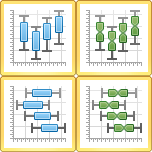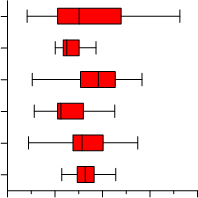# Box Plots

 Click the Home | New Graph | Statistical | Vertical Box Plot or Home | New Graph | Statistical | Notched Vertical Box Plot command to show the minimum, maximum, median, lower quartile, and upper quartile for a particular X group.   Click the Home | New Graph | Statistical | Horizontal Box Plot or Home | New Graph | Statistical | Notched Horizontal Box Plot command to show the minimum, maximum, median, lower quartile, and upper quartile for a particular Y group.   Box plot and notched box plot orientation cannot be changed once the plot is created. You must delete the existing plot and create the desired plot to change the box plot orientation, e.g. from Vertical Box Plot to Horizontal Box Plot.   The caps, or whiskers, at the end of each box indicate the extreme values (minimum and maximum, inter-quartile range times a factor, or a percentage of the data), the box is defined by the lower and upper quartiles, and the line in the center of the box is the median. The values are sorted before referencing.Click the Vertical Box Plot; Notched Vertical Box Plot; Horizontal Box Plot; or Notched Horizontal Box Plotbuttons to create box and whisker plots.Box plots show extreme values, median, lower quartile, and

upper quartile information for groups of data.

In addition, the box plot options let you display symbols for each of the outlier samples in your data group. The definitions of these terms are:

 Term Definition Median For an odd number of data points, this is the middle value. For an even number points, it is the mean of the middle values. First Quartile Value[(0.25 x (number of data points) + 0.5, rounded off to nearest whole number] Third Quartile Value[0.75 x (number of data points) + 0.5, rounded off to nearest whole number]

## Creating a New Box Plot

To create a 2D box-whisker plot:

1. Click the Home | New Graph | Statistical | Vertical Box Plot;Home | New Graph | Statistical | Notched Vertical Box Plot; Home | New Graph | Statistical | Horizontal Box Plot; or Home | New Graph | Statistical | Notched Horizontal Box Plot command

2. Select a data file in the Open Worksheet dialog. You can select a new data file or you can select an open data file in the Open worksheets section.

3. Click the Open button. A box-whisker plot is created using the default properties.

## Editing Box Plot Properties

To change the features of a box-whisker plot— including the columns used to create the plot— first select the box plot in the plot window or Object Managerand then edit its properties in the Property Manager.

Click the following tabs in the Property Manager to change different properties: